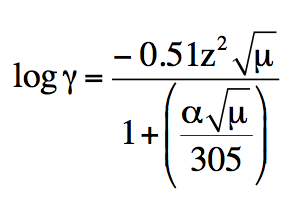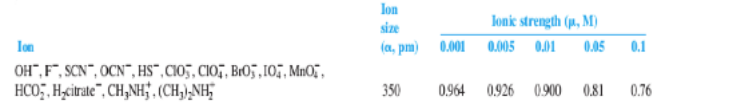Start typing, then use the up and down aroows to select an option from the list.

# Analytical Chemistry

Learn the toughest concepts covered in your Analytical Chemistry class with step-by-step video tutorials and practice problems.

Next ChapterActivity and the Systematic Treatment of Equilibrium

Activity coefficients is factor used in describing the departure from ideal behavior for a reaction mixture.

## Activity Coefficients

1

### concept

Activity Coefficient5m
Play a video:
0
2

### example

Activity Coefficient1m
Play a video:
0
3

### example

Activity Coefficient1m
Play a video:
0

## Activity Coefficient Table

4

### example

Activity Coefficient Table

The video is coming soon
0
5

### example

Activity Coefficient Table Calculations 1

The video is coming soon
0
6

### example

Activity Coefficient Table Calculations 12m
Play a video:
0
7
Problem

Calculate the activity coefficient of H+ using the extended Debye-Huckel equation for a solution comprised of H+ and I . Given that H+ has a size of 9.00 x 10-10 m and the molar concentration of the solution is 0.075.

log γ = − 0.51 z 2 µ / 1 + (α µ / 305)## Interpolation: Non-ideal Ionic Strength

8

### concept

Non-Ideal Ionic Strength

The video is coming soon
0
9

### example

Non-Ideal Ionic Strength

The video is coming soon